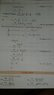# Please explain this limit problem

rishi kesh
OP warned about not using the homework template
Please explain the above limit problem.i am able to understand last steps but can't get initial 4 steps.

#### Attachments

•1513776043655-874305.jpg
21.3 KB · Views: 414

Homework Helper
Gold Member
The sum of natural numbers 1 to N. Is that the part you are confused about?
Let's do an example. Say you want to add the numbers 1 to 10. You can group them: Take the first number and the last number (1 + 10) then 2nd number and next to last (2 + 9), then (3 + 8) etc. Each grouping adds to 11 {N+1}. There are 5 groupings {N/2}. So in general, we have (N + 1) * (N/2). This works with odd N values as well.

Last edited:
•rishi kesh
rishi kesh
The sum of natural numbers 1 to N. Is that the part you are confused about?
Let's do an example. Say you want to add the numbers 1 to 10. You can group them: Take the first number and the last number (1 + 10) then 2nd number and next to last (2 + 9), then (3 + 8) etc. Each grouping adds to 11. There are 5 groupings. So in general, we have (N + 1) * (N/2). This works with odd N values as well.
This trick is something interesting to know about. I will remember it.appreciate your help•scottdave
rishi kesh
This trick is something interesting to know about. I will remember it.appreciate your helpBut of you look at the 4th step they replaced 'n' by n-1 ..how do that work?

Mentor
But of you look at the 4th step they replaced 'n' by n-1 ..how do that work?
The comment appears to be misplaced. The replacement occurs one step above, going from ##\sum_{r=1}^n## to ##\sum_{r=1}^{n-1}##.

rishi kesh
The comment appears to be misplaced. The replacement occurs one step above, going from ##\sum_{r=1}^n## to ##\sum_{r=1}^{n-1}##.
Oh i got it. :)